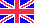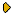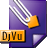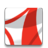Журнал Экспериментальной и Теоретической Физики
 НАЧАЛО | ПОИСК | ДЛЯ АВТОРОВ | ПОМОЩЬОбщая информация о журналеЗолотые страницыАдреса редакцииСодержание журналаСообщения редакцииПравила для авторовЗагрузить статьюПроверить статус статьиЖЭТФ, Том 135, Вып. 3, стр. 419 (Март 2009)
(Английский перевод - JETP, Vol. 108, No 3, p. 365, March 2009 доступен on-line на www.springer.com )

BOUND STATES AND SCATTERING LENGTHS OF THREE TWO-COMPONENT PARTICLES WITH ZERO-RANGE INTERACTIONS UNDER ONE-DIMENSIONAL CONFINEMENT

Поступила в редакцию: 19 Августа 2008

PACS: 31.15.ac, 03.65.Ge, 34.50.-sDJVU (121.7K)PDF (355.7K)

The universal three-body dynamics in ultracold binary gases confined to one-dimensional motion is studied. The three-body binding energies and the (2+1)-scattering lengths are calculated for two identical particles of mass m and a different particle of mass m1, whose interaction is described in the low-energy limit by zero-range potentials. The critical values of the mass ratio m/m1 at which three-body states occur and the (2+1)-scattering length vanishes are determined for both zero and infinite interaction strength λ1 of the identical particles. A number of exact results are listed and asymptotic dependences for both m/m1 → ∞ and λ1 → -∞ are derived. Combining the numerical and analytic results, we deduce a schematic diagram showing the number of three-body bound states and the sign of the (2+1)-scattering length in the plane of the mass ratio and the interaction-strength ratio. The results provide a description of the homogeneous and mixed phases of atoms and molecules in dilute binary quantum gases.

Сообщить о технических проблемах